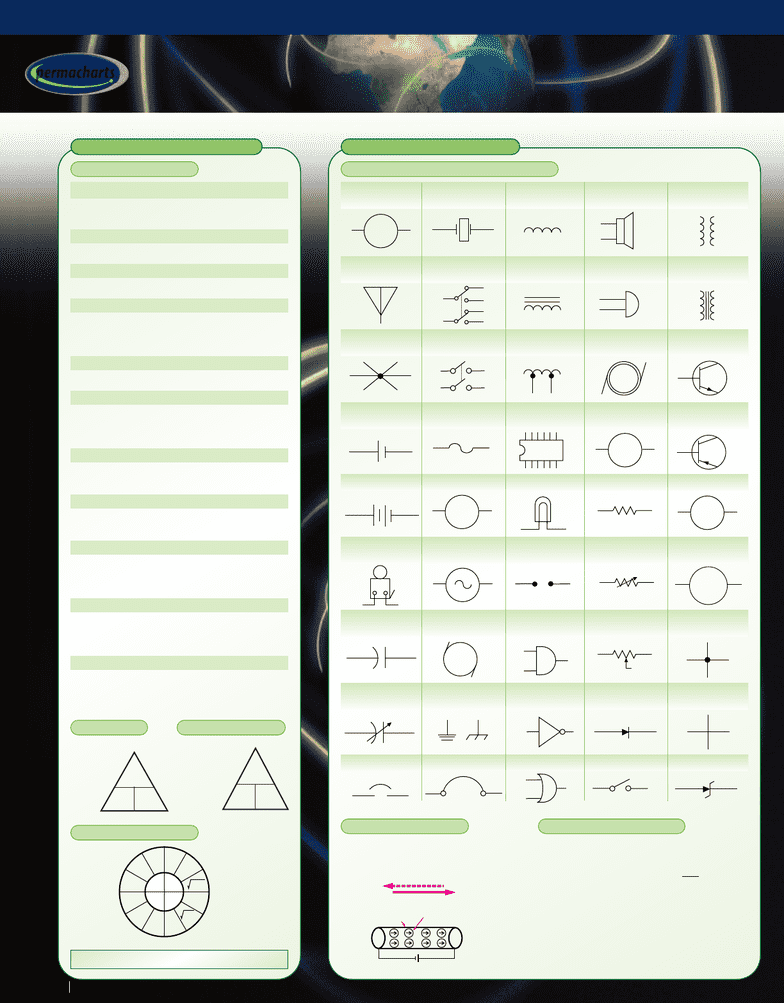Get 2 days of unlimited access
Class Notes (1,000,000)
US (420,000)
UA (100)
4400 (5)
All (5)
Reference Guide

# Electricity & Magnetism - Reference Guides

Department
Electrical Engineering
Course Code
4400:307
Professor
All
Chapter
Permachart

This preview shows page 1. to view the full 4 pages of the document.ELECTRICITY B A S I C S
Electricity & Magnetism
Electricity & Magnetism
FUNDAMENTAL TERMS
Potential difference (Vor E):
Charges interacting with each other to do
work by attraction or repulsion • Unit: volt (V)
Electric charge (Q or q):
Unit of electricity • Unit: coulomb (C)
Electric current (I):
Measure of electron flow • Unit: ampere (A)
Resistance (R):
Measure of opposition to current flow
• Found from Ohm’s Law; R = V/I
• Unit: ohm (Ω)
Electric energy (E):
E = VIt = Pt • Unit: joule (J), electron-volt (eV)
Electric power (P):
Rate of electric energy • P = VI, or P = V2/R,
or P = I2R• Unit: watt (W) or J/s, horsepower
(hp)
Frequency (f):
Cycles/second • f = 1/T
• Unit: hertz (Hz or s–1)
Capacitance (C):
Ability to store electric charge
• Unit: farad (F)
Inductance (L):
Ability of a conductor to induce voltage in
itself when the current changes
• Unit: henry (H)
Capacitive reactance (XC):
Opposition to flow of AC current due to
capacitance in circuit • XC= 1/(2πfC)
• Unit: ohm (Ω)
Inductive reactance (XL):
Opposition to flow of AC current due to
inductance in circuit • XL= 2πfL
• Unit: ohm (Ω)
ELECTRIC C I R C U I T S
CIRCUIT SYMBOLS FOR SCHEMATIC DIAGRAMS
CURRENT FLOW
• Conventional flow is used in
North America; current flow is
used in Europe
OHMS LAW
• V = IR
I= V/R
• R = V/I
MEMORY AID
POWER TRIANGLE
• P = VI
• V = P/I
• I = P/V
VR
V
ELECTRIC POTENTIAL ENERGY
• Energy between two point charges
separated a distance, r(or d)
(i) Ueis electric
potential energy,
kis Coulomb’s constant
(ii) q1& q2are point charges
(iii) For like charges, Ueis positive for a finite
distance r
PI
RV
I2R
VI V/R
P/V
V/I
P/R
IR
PR
P/I
V2/R
V2/P
P/I2
Ammeter
Antenna
Arc Lamp
Battery Cell
Battery
Buzzer
Capacitor
(fixed)
Capacitor
(variable)
Circuit Breaker
Crystal
DPDT Switch
DPST Switch
Fuse
Galvanometer
Generator (AC)
Generator
(DC)
Ground
Inductor
(air-core)
Inductor
(iron-core)
Inductor
(tapped)
Integrated
Circuit
Lamp
Lightning
Arrestor
Logic AND
Gate
Logic Inverter
Gate
Logic OR Gate
Loudspeaker
Microphone
Motor (AC)
Motor (DC)
Resistor (fixed)
Resistor
(variable)
Rheostat
Semiconductor
Diode
Switch
Transformer
(general)
Transformer
(iron-core)
Transistor
(NPN)
Transistor
(PNP)
Voltmeter
Wattmeter
Wires
(connected)
Wires
(unconnected)
Zener Diode
A
G
or
W
V
BC
E
Conductor Free electrons
in motion
+Q
-Q
E
Electron flow
Conventional flow
Uk
qq
r
e=12
BC
E
+
+
RI I
Note: Some sources use Ein place of V
ELECTRICITY & MAGNETISM • A-817-61
l e a r n r e f e r e n c e r e v i e w
TM
permacharts
© 1998-2013 Mindsource Technologies Inc.
www.permacharts.com
###### You're Reading a Preview

Unlock to view full version